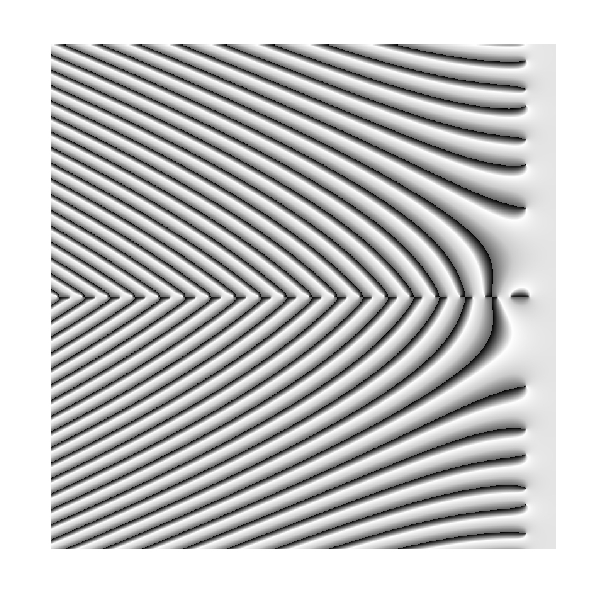Rhombuses

For a lonely soul, you’re having such a nice time (Nothing in my way, Keane)

In my previous post, I created the P2 Penrose tessellation according to the instructions of this post. Now it’s time to create the P3 tessellation following the same technique I described already. This is the image of the P3 tessellation:

Note that all tiles are rhombuses. I recognize that I like the P3 more than the P2, I do not really why. What about you? Here you have the code to play with it if you want.

The Zebra Of Riemann

Mathematics is the art of giving the same name to different things (Henri Poincare)

Many surveys among experts point that demonstration of the Riemann Hypothesis is the most important pending mathematical issue in this world. This hypothesis is related to Riemann zeta function, which is supossed to be zero only for those complex whose real part is equal to 1/2 (this is the conjecture of Riemann itself). Confirming the conjecture would imply deep consequences in prime numbers teory and also in our knowledge of their properties. Next plot represents the argument of zeta function over complex with real part between -75 and 5 (x axis) and imaginary part between -40 and 40 (y axis). An explanation of this kind of graph can be found here. Does not it remind you of something?Solving the hypothesis of Riemann is one of the seven Clay Mathematics Institute Millennium Prize Problems. Maybe zebras keep the secret to solve it in their skins.

This is the code to plot the graph:

require(pracma)
z=outer(seq(-75, 5, by =.1),1i*seq(-40, 40, by =.1),'+')
z=apply(z, c(1,2), function(x) Arg(zeta(x)))
opt=theme(legend.position="none",
panel.background = element_blank(),
panel.grid = element_blank(),
axis.ticks=element_blank(),
axis.title=element_blank(),
axis.text =element_blank())
z=data.frame(expand.grid(x=seq(ncol(z)), y=seq(nrow(z))), z=as.vector(z))
require(ggplot2)
ggplot(z, aes(x=x, y=y, color=z)) + geom_tile() + scale_colour_gradientn(colours=c("black","white", "gray80", "gray40")) + opt